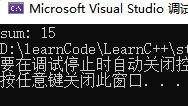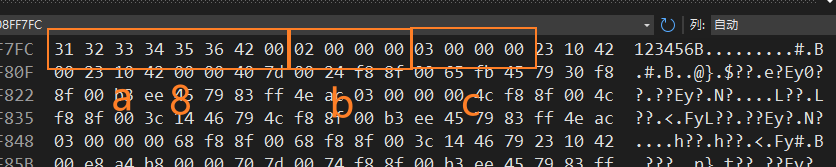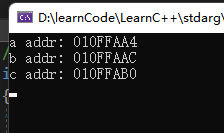## 引言

C语言虽然不支持泛型编程（至少C98是这样的），但是C语言却支持不定参数的函数，这里我深究一下里面的原理，并且学会它的使用，自己简单实现一个简单的printf函数。 注：这里使用的IDE为 vs2022

    _Check_return_opt_
_CRT_STDIO_INLINE int __CRTDECL printf(
_In_z_ _Printf_format_string_ char const* const _Format,
...)


## C语言 stdarg.h

### 定义

void va_start(va_list ap, last_arg)这个宏初始化 ap 变量，使ap指向起始的参数(last_arg)
type va_arg(va_list ap, type)获取下一个类型为type的参数
void va_end(va_list ap)释放ap
va_copy(destination, source)拷贝ap的内容

### 示例

#include<stdio.h>
#include<stdarg.h>
//实现 args_nums个int类型的数相加
int sum(int args_num, ...)
{
va_list ap;
int sum = 0;
va_start(ap, args_num);//1. 初始化 ap

for (; args_num > 0; --args_num)
sum += va_arg(ap, int);//2. 获取 下一个int类型的参数
va_end(ap);//结束使用
return sum;
}
int main()
{
printf("sum: %d", sum(5, 1, 2, 3, 4, 5));
return 0;
}


1. va_start(ap, args_num) 初始化ap
2. va_arg(ap, int) 获取下一个int类型参数
3. va_end(ap) 结束使用
4. 补充：stdarg.h 并没有提供帮我们判断不定参数有多个的方法，这里我是用 传入一个args_num来标记有多个不定参数，不要以为我们必须传入一个int来标记，我们可以采取其他方法的（后面补充）
5. 这里我们必须传入一个确定的参数作为第一个参数，因为 va_start 需要一个确定的参数初始化## ta的原理

### 函数传参数的本质

C语言是最接近汇编的一门语言，函数传参的本质到底是什么，简单一句话 ——将参数压栈，如何你有汇编的经历的话，就知道如果要给一个过程传入参数就需要你提前将传入的参数压入栈中，C语言就是这样做的，当然控制压栈这么麻烦的操作编译器在编译的过程中就帮你完成了。当然这要拿出汇编中的一个知识点，每次压栈和出栈的基本单位不是字节，而是当前CPU的字长为单位的，比如 32位那么每次压栈就是以4字节位基本单位的。

#define VNAME(val) (#val) // 获取val变量的名字
typedef struct test { char c; } test; //定义结构体 test
void foo(test a, char b,int c )
{
}

int main()
{
test t = { "123456" };
foo(t, 2, 3);
return 0;
}char* p = &a;
char bb = *(char*)(p += 8);
int cc = *(int*)(p += 4);
printf("b : %d,c : %d\n", bb, c);


    typedef char* va_list;   //和我想得一样
#define _INTSIZEOF(n)          ((sizeof(n) + sizeof(int) - 1) & ~(sizeof(int) - 1)) //求n类型 在栈中所占空间
#define __crt_va_start_a(ap, v) ((void)(ap = (va_list)_ADDRESSOF(v) + _INTSIZEOF(v))) //初始化 ap
#define __crt_va_arg(ap, t)     (*(t*)((ap += _INTSIZEOF(t)) - _INTSIZEOF(t)))//获取下一个变量
#define __crt_va_end(ap)        ((void)(ap = (va_list)0))//结束使用ap
#define va_copy(destination, source) ((destination) = (source))//拷贝


### _INTSIZEOF(n)

#define _INTSIZEOF(n)          ((sizeof(n) + sizeof(int) - 1) & ~(sizeof(int) - 1))


_INTSIZEOF(n)整个做的事情就是将n的长度化为int长度的整数倍,比如n为5，二进制就是101b，int长度为4，二进制为100b，那么n化为int长度的整数倍就应该为8。换一句话说它就帮我们求出变量间的偏移量。

~是位取反的意思。~(sizeof(int) – 1) )就应该为~（4-1）=~（00000011b）=11111100b，这样任何数& ~(sizeof(int) – 1) )后最后两位肯定为0，就肯定是4的整数倍了。(sizeof(n) + sizeof(int) – 1)就是将大于4m但小于等于4（m+1）的数提高到大于等于4（m+1）但小于4(m+2)（m为0,1,2,…），这样再& ~(sizeof(int) – 1) )后就正好将原长度补齐到4的倍数了。

MSVC是这样实现的，我们可以看看 GNUC是如何实现的：

#define __va_rounded_size(TYPE)  \  //名字虽然不一样但是功能是一样的，毕竟这是不同的厂家
(((sizeof (TYPE) + sizeof (int) - 1) / sizeof (int)) * sizeof (int))


## 练习 实现printf

#include<stdio.h>
#include<stdarg.h>
int myPrintf(const char* string, ...);
int main()
{
//printf("sum: %d", sum(5, 1, 2, 3, 4, 5));
myPrintf("helloworld%d%s%c\na", 15, "122", 'z');
return 0;
}
//大致思路 将string 中的 %d %c \n 等 替换成 对应的字符 存储再 buffer中
//这里只实现了 %d %c %s \n
int myPrintf(const char* string, ...)//返回实际输出字符个数
{

char buffer[BUFSIZ];

int temp = 0;
va_list arg;

char* p_string = NULL;
char* p_buffer = buffer;
char* p_temp = NULL;

int counter = 0;//输出字符长度
int number = 0;
int foo = 0;

va_start(arg, string);

for (counter = 0, p_string = string; *(p_string) != '\0';)
{
switch (*p_string)
{
//处理 %
case '%':
{
switch (*++p_string)
{
case 'd':

temp = va_arg(arg, int);

foo = temp;
//获取 位数
while (foo)
{
number++;
counter++;
foo /= 10;
}

foo = temp;
//整数转字符串
while (number)
{
*(p_buffer + number - 1) = (foo % 10)+'0';
foo /= 10;
number--;
}

p_buffer += number;
break;

case 'c':
temp = va_arg(arg, int);
*(p_buffer++) = temp;
break;

case 's':
p_temp = va_arg(arg, char*);

while (*p_temp !='\0')
{
*(p_buffer++) = *(p_temp++);
counter++;
}
break;

default:
break;

}
++p_string;
break;
}
//处理 转移字符
case '\\':
{
p_string++;
switch (*p_string)
{
case 'n':
*(p_string++) = '\n';
break;
default:
break;
}
break;
}
default:
*(p_buffer++) = *(p_string++);
counter++;
}
}
*(p_buffer++) = '\0';
va_end(arg);
p_buffer = NULL;

puts(buffer);
return counter;
}


## 结语

C语言处理这类问题，非常底层，看似简单，如果你是一个新手，那么报错越界是时常发生的事情。那么C++处理这种问题,又是啥操作呢？。